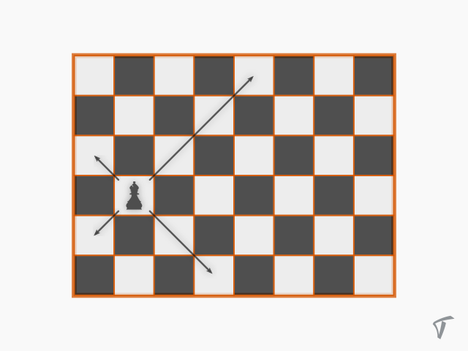# Practice on Toph

Participate in exhilarating programming contests, solve unique algorithm and data structure challenges and be a part of an awesome community.

# Gutibaji on Chessboard

By himuhasib · Limits 1s, 512 MB

Let there be a chessboard of size $N \times M$, and only one bishop in a cell $(x, y)$. How many cells can the bishop can attack in one move? You can assume that one possible move is staying in the current position.## Input

In the first line of input, there will be a number $T$ ($1 ≤ T ≤ 1000$) denoting the number of test cases.

On the next $T$ lines, there will be four integers $N$, $M$, $x$, $y$ as described above. Here $N$ ($1 ≤ N ≤ 10^{12}$) is the number of columns and $M$ ($1 ≤ M ≤ 10^{12}$) is the number of rows. The bishop is at column $x$ ($1 ≤ x ≤ N$), row $y$ ($1 ≤ y ≤ M$).

## Output

For each test case print a single line with the number of cells the bishop can attack with one move.

## Sample

InputOutput
2
8 6 2 4
8 6 1 1
8
6


The image above is a visualization of the first case.MathuDebug

### Statistics

79% Solution Ratio

ruhanhabib39Earliest, Dec '16

abdullah2222Fastest, 0.0s

ruhanhabib39Lightest, 131 kB

mdvirusShortest, 125B

### Submit

 Tough Cubs' Contest, December 2016Dec '16 SUST Intra University Programming Contest 2017 (Junior) Selection 3Jul '17